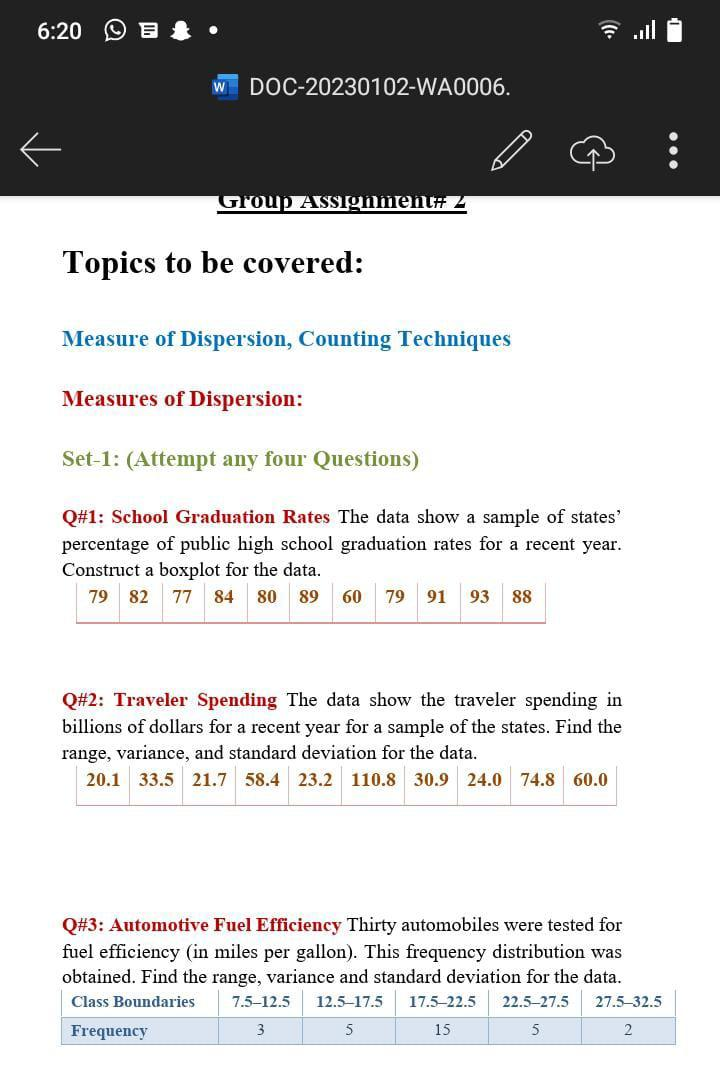Home / Expert Answers / Statistics and Probability / topics-to-be-covered-measure-of-dispersion-counting-techniques-measures-of-dispersion-set-1-a-pa935

# (Solved): Topics to be covered: Measure of Dispersion, Counting Techniques Measures of Dispersion: Set-1: (A ...Topics to be covered: Measure of Dispersion, Counting Techniques Measures of Dispersion: Set-1: (Attempt any four Questions) Q\#1: School Graduation Rates The data show a sample of states' percentage of public high school graduation rates for a recent year. Construct a boxplot for the data. Q\#2: Traveler Spending The data show the traveler spending in billions of dollars for a recent year for a sample of the states. Find the range, variance, and standard deviation for the data. Q\#3: Automotive Fuel Efficiency Thirty automobiles were tested for fuel efficiency (in miles per gallon). This frequency distribution was obtained. Find the range, variance and standard deviation for the data.

We have an Answer from Expert